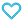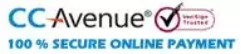# Objective NCERT Question Bank for JEE-Mathematics

600.00

Discount offer on this book in a bundle, click to view

Objective NCERT Question Bank Mathematics accommodates Boards (Term I & Term II) and JEE like objective questions at your fingertips, extracted from NCERT Mathematics Text Books of class 11th and 12th. Practicing such questions in an exam like environment will gauze your performance in actual exams. A self-analysis meter to check your performance along with answer key, hints and explanations are given at the end of every chapter. NCERT Exemplar problems and Assertion Reason Corner are included in the book. This gives you complete practice and preparation not only of the important questions of the NCERT but also the understanding of type of questions asked in Boards and JEE entrance exams.

985 in stock

Qty:Add to WishlistObjective NCERT Question Bank Mathematics accommodates Boards (Term I & Term II) and JEE like objective questions at your fingertips, extracted from NCERT Mathematics Text Books of class 11th and 12th. Practicing such questions in an exam like environment will gauze your performance in actual exams. A self-analysis meter to check your performance along with answer key, hints and explanations are given at the end of every chapter. NCERT Exemplar problems and Assertion Reason Corner are included in the book. This gives you complete practice and preparation not only of the important questions of the NCERT but also the understanding of type of questions asked in Boards and JEE entrance exams.

CONTENT:
• Class XI
• 1. Sets
• 2. Relations and Functions
• 3. Trigonometric Functions
• 4. Principle of Mathematical Induction
• 5. Complex Numbers & Quadratic Equations
• 6. Linear Inequalities
• 7. Permutations and Combinations
• 8. Binomial Theorem
• 9. Sequences and Series
• 10. Straight Lines
• 11. Conic Sections
• 12. Introduction to Three Dimensional Geometry
• 13. Limits and Derivatives
• 14. Mathematical Reasoning
• 15. Statistics
• 16. Probability
• Class XII
• 1. Relations and Functions
• 2. Inverse Trigonometric Functions
• 3. Matrices
• 4. Determinants
• 5. Continuity and Differentiability
• 6. Application of Derivatives
• 7. Integrals
• 8. Application of Integrals
• 9. Differential Equations
• 10. Vector Algebra
• 11. Three Dimensional Geometry
• 12. Linear Programming
• 13. Probability
• Practice papers
• Practice Paper - 1
• Practice Paper - 2
• Practice Paper - 3
• Practice Paper - 4
• Practice Paper - 5
ISBN11 9789355550361 MTG Editorial Board 2021-22 680 Class 11, Class 12 Regional Ent. Exams-Engg Mathematics 990gm

## Reviews

There are no reviews yet.